#### 期刊菜单

Research on the Method of Monocular Camera Calibration
DOI: 10.12677/JISP.2023.121004, PDF, HTML, XML, 下载: 37  浏览: 123

Abstract: In order to reduce the error and improve the calibration accuracy, the calibration parameters obtained by the solution are nonlinear iteratively based on the plane checkerboard calibration method to minimize the reprojection error and find the global optimal solution. After analyzing the theoretical imaging model of the camera and studying the nonlinear camera imaging model after introducing distortion parameters, the camera transformation matrix and the parameters in the camera are solved, and finally the calibration results are optimized. The experimental results show that the mean calibration error of this method is 0.1402 and the standard deviation is 0.0207, which has higher accuracy and better stability than the traditional calibration method and has certain engineering practical significance.

1. 引言

2. 相机标定模型与方法

2.1. 相机模型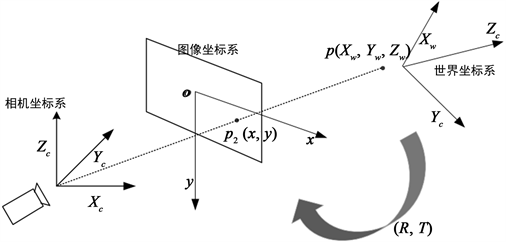Figure 1. Camera coordinate system conversion diagram

$\frac{{X}_{c}}{x}=\frac{{Z}_{c}}{f}=\frac{{Y}_{c}}{y}$ (1)

$x=f\frac{{X}_{c}}{{Z}_{c}},y=f\frac{{Y}_{c}}{{Z}_{c}}$ (2)

${Z}_{c}\left[\begin{array}{c}x\\ y\\ 1\end{array}\right]=\left[\begin{array}{cccc}f& 0& 0& 0\\ 0& f& 0& 0\\ 0& 0& 1& 0\end{array}\right]\left[\begin{array}{c}{X}_{c}\\ {Y}_{c}\\ {Z}_{c}\\ 1\end{array}\right]$ (3)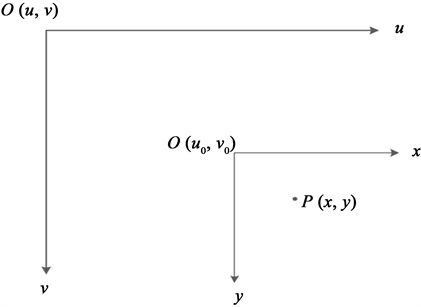Figure 2. Pixel coordinate system conversion diagram

$\left\{\begin{array}{l}u=\frac{x}{dx}+{u}_{0}\\ v=\frac{y}{dy}+{v}_{0}\end{array}$ (4)

$\left[\begin{array}{c}u\\ v\\ 1\end{array}\right]=\left[\begin{array}{ccc}\frac{1}{dy}& 0& {u}_{0}\\ 0& \frac{1}{dy}& {v}_{0}\\ 0& 0& 1\end{array}\right]\left[\begin{array}{c}x\\ y\\ 1\end{array}\right]$ (5)

$\left[\begin{array}{c}{X}_{c}\\ {Y}_{c}\\ {Z}_{c}\end{array}\right]=R\left[\begin{array}{c}{X}_{w}\\ {Y}_{w}\\ {Z}_{w}\end{array}\right]+T$ (6)

$\left[\begin{array}{c}{X}_{c}\\ {Y}_{c}\\ {Z}_{c}\\ 1\end{array}\right]=\left[\begin{array}{cc}R& T\\ 0& 1\end{array}\right]\left[\begin{array}{c}{X}_{w}\\ {Y}_{w}\\ {Z}_{w}\\ 1\end{array}\right]$ (7)

${Z}_{c}\left[\begin{array}{c}u\\ v\\ 1\end{array}\right]=\underset{相机内参}{\underset{︸}{\left[\begin{array}{cccc}{f}_{x}& 0& {u}_{0}& 0\\ 0& {f}_{y}& {v}_{0}& 0\\ 0& 0& 1& 0\end{array}\right]}}\underset{相机外参}{\underset{︸}{\left[\begin{array}{cc}{R}_{3×3}& {T}_{3×1}\\ 0& 1\end{array}\right]}}\left[\begin{array}{c}{X}_{w}\\ {Y}_{w}\\ {Z}_{w}\\ 1\end{array}\right]$ (8)

2.2. 畸变模型

$\left(u,v\right)$ 为理想无畸变的像素坐标， $\left(\stackrel{⌣}{u},\stackrel{⌣}{v}\right)$ 式实际观测到的像素坐标。 $\left(\stackrel{⌣}{x},\stackrel{⌣}{y}\right)$$\left(x,y\right)$ 为实时有畸变和理想无畸变的图像坐标，将式(9)代入可构建畸变模型式(10)。

$\left\{\begin{array}{l}\stackrel{⌣}{u}={u}_{0}+\alpha \stackrel{⌣}{x}+\gamma \stackrel{⌣}{y}\\ \stackrel{⌣}{v}={v}_{0}+\beta \stackrel{⌣}{y}\end{array}$ (9)

$\left\{\begin{array}{l}\stackrel{⌣}{u}=u+\left(u-{u}_{0}\right)\left[{k}_{1}\left({x}^{2}+{y}^{2}\right)+{k}_{2}{\left({x}^{2}+{y}^{2}\right)}^{2}\right]+{p}_{1}\left(3{x}^{2}+{y}^{2}\right)+2{p}_{2}xy\\ \stackrel{⌣}{v}=v+\left(v-{v}_{0}\right)\left[{k}_{1}\left({x}^{2}+{y}^{2}\right)+{k}_{2}{\left({x}^{2}+{y}^{2}\right)}^{2}\right]+{p}_{2}\left({x}^{2}+3{y}^{2}\right)+2{p}_{1}xy\end{array}$ (10)

2.3. 非线性优化

$\mathrm{min}\underset{i=1}{\overset{n}{\sum }}\underset{j=1}{\overset{m}{\sum }}{‖{m}_{i}{}_{j}-\stackrel{˜}{m}\left({R}_{i},{t}_{i},k,p,{M}_{ij}\right)‖}^{2}$ (11)

$err=\frac{1}{mn}\mathrm{min}\underset{i=1}{\overset{n}{\sum }}\underset{j=1}{\overset{m}{\sum }}{‖{m}_{i}{}_{j}-\stackrel{˜}{m}\left({R}_{i},{t}_{i},k,p,{M}_{j}\right)‖}_{2}^{1/2}$ (12)

3. 实验结果与分析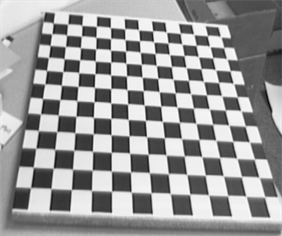(a) 标定板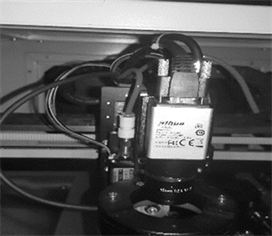(b) 工业相机

Figure 3. Calibration plate and camera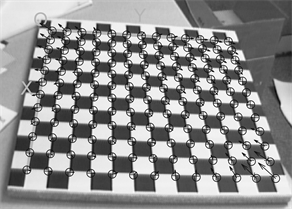Figure 4. Calibration plate corner plotTable 1. Camera internal references and distortion coefficients

${T}_{1}=\left[\begin{array}{cccc}0.9921& 0.5231& 0.1136& -147.5905\\ 0.0548& 0.6351& -0.7705& -71.3822\\ -0.1125& -0.7707& 0.6272& 710.6725\\ 0& 0& 0& 1\end{array}\right]$

${T}_{2}=\left[\begin{array}{cccc}0.9927& 0.0566& 0.1068& -128.7775\\ 0.0054& 0.8621& -0.5066& -134.2187\\ -0.1208& -0.5035& 0.8555& 630.8953\\ 0& 0& 0& 1\end{array}\right]$

${T}_{3}=\left[\begin{array}{cccc}0.9857& 0.1661& 0.0301& -103.9663\\ -0.1585& 0.8497& -0.5029& -146.9009\\ -0.0580& -0.5005& 0.8638& 645.7499\\ 0& 0& 0& 1\end{array}\right]$

${T}_{4}=\left[\begin{array}{cccc}0.9015& 0.0030& 0.4328& -53.3639\\ 0.2251& 0.5874& -0.7774& -130.5077\\ -0.3497& -0.5443& 0.5853& 648.7138\\ 0& 0& 0& 1\end{array}\right]$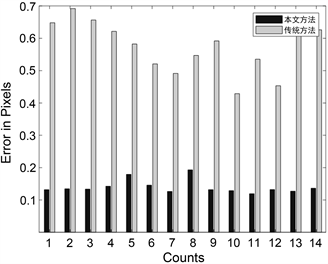Figure 5. Calibration error comparison chartTable 2. Coordinate error result analysis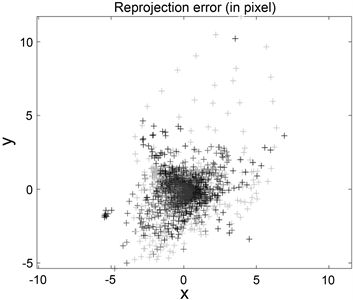Figure 6. Reprojection error map

4. 结论

  Meng, X. and Hu, Z. (2003) A New Easy Camera Calibration Technique Based on Circular Points. Pattern Recognition, 36, 1155-1164. https://doi.org/10.1016/S0031-3203(02)00225-X  Bu, L., Huo, H., Liu, X., et al. (2021) Concentric Circle Grids for Camera Calibration with Considering Lens Distortion. Optics and Lasers in Engineering, 140, 106527. https://doi.org/10.1016/j.optlaseng.2020.106527  Shi, Z.C., Shang, Y., Zhang, X.F., et al. (2021) DLT-Lines Based Camera Calibration with Lens Radial and Tangential Distortion. Experimental Mechanics, 61, 1237-1247. https://doi.org/10.1007/s11340-021-00726-5  林旭东, 张旭. 基于透视畸变矫正的高精度相机标定方法[J]. 激光与光电子学进展, 2022: 1-15.  孙聪, 杨正磊, 贾萌娜, 于起峰. 基于圆锥曲线和直线融合特征的长焦相机标定方法[J]. 光学学报, 2022, 42(12): 185-197.  刘艳, 李腾飞. 对张正友相机标定法的改进研究[J]. 光学技术, 2014, 40(6): 565-570.  Zhang, Z. (2000) A Flexible New Technique for Camera Calibration. IEEE Transactions on Pattern Analysis and Machine Intelligence, 22, 1330-1334. https://doi.org/10.1109/34.888718  赵敏, 李晓港, 苏泽鹏, 朱凌建. 基于定向分层靶标的相机参数标定[J]. 光学学报, 2022, 42(16): 144-152.  吴军, 张美妙, 刘少禹, 祝玉恒, 袁少博. 基于旋转标定板的多相机系统标定方法[J]. 激光与光电子学进展, 2022, 59(17): 180-189.  翟溢章, 宿洁华, 张士恒, 吴恩启. 相机标定的精度影响实验分析[J]. 电子设计工程, 2022, 30(7): 82-86.  施佳豪, 王庆, 冯悠扬. 基于三维标定板的相机标定方法[J]. 传感器与微系统, 2021, 40(6): 48-51.